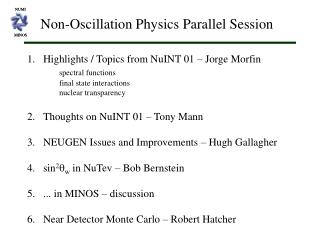Download PresentationNUMI

# NUMI - PowerPoint PPT PresentationDownload Presentation## NUMI

- - - - - - - - - - - - - - - - - - - - - - - - - - - E N D - - - - - - - - - - - - - - - - - - - - - - - - - - -
##### Presentation Transcript

1. NUMI MINOS Non-Oscillation Physics Parallel Session • Highlights / Topics from NuINT 01 – Jorge Morfin • spectral functions • final state interactions • nuclear transparency • Thoughts on NuINT 01 – Tony Mann • NEUGEN Issues and Improvements – Hugh Gallagher • sin2qw in NuTev – Bob Bernstein • ... in MINOS – discussion • Near Detector Monte Carlo – Robert Hatcher

2. Some time ago it was noticed that the resonance production • cross section exhibited some strange features: • s proportional to E at high energies • s(nt) = s(nm) at high energies • s(nt)/s(nm) too high at low energies Resonance Production Resonance / single pion production: Rein-Seghal model. Uses the Feynman-Kivlinger-Ravndal model of the nucleon to solve explicitly for the bound states. These are then identified with the measured resonances and the transition amplitudes are calculated directly. 17 resonances in the model, predictions dominated by the D(1232) at low energies. The Table at right gives the cross sections for n n m R+ Units are 10-38 cm2 (from Annals of Physics, 133, (1981) 79) Other MC codes use different models, some involve no resonance production other than the D. Does the available data show evidence of non-D(1232) resonance production?

3. Resonance Production A previous kludge had been to just eliminate all n=2 resonances from the calculation. n=1 n=2 original with W cut n=0 no n=2 Resonances now only contribute for W<2 GeV.

4. Resonance Production s(nt)/s(nm) too high at low energies s(5GeV) ~ 3 times the QEL cross section Resonance The lepton mass terms are accounted for in the calculation of phase space but not in the matrix element. QEL Include a fudge factor which is the ratio of the QEL matrix elements with and without the tau mass included. DIS

5. + propagator term (1+Q2/mA2)-2 n n p0 Z N N P Coherent Production The cross section for neutral current coherent production has been included according to the calculation of Rein and Seghal NPB223 (1983) 29. (PCAC prediction) Ansatz With mA=1 GeV

6. nm n p p+ p- total resonance Generator Evaluation Tools 300 \\ number of bins2 \\ ptype: 1=total, 2 = differential0. \\ ptype = 1 -> emin40. \\ emax20. \\ ptype = 2 -> e_val2 \\ kvar = plot variable1 \\ fluxid2 \\ iwant = plot range (1=auto, 2 = select)1. \\ iwant = 2 -> kmin3. \\ kmax7 \\ nutyp1 \\ ccnc_want: (1=cc, 2=nc, 3=both)0 \\ target: (nucleus / particle code, -1 for isoscalar)10110 \\ ifinal: (of the form pn+-0)2 \\ im_want: (1=nu-p, 2=nu-n, 3=nubar-p, 4=nubar-n)13 \\ process_mask: (bits for qel,res,dis,coh)0 \\ icuts: cuts variable (0=none, 1=abs(qqs), 2=w, 3=x, 4=y)0. \\ icuts>0 -> cutmin1.6 \\ cutmax0 \\ qelsum: >0 means sum add qel channel0 \\ ressum: >0 means sum all resonances channels0 \\ dissum: >0 means sum all dis 1 I have written an independent program that calls the differential cross section routines for the various processes to display total or differential cross sections. • Specify cuts on kinematic variables • Select specific processes • Weight differential distributions by fluxes for ANL, • BNL WBB, Gargamelle (PS), or BEBC (WA-25). • Requires a full determination of inclusive -> exclusive • fragmentation functions.

7. s(n + N m+ +Y*) = tan2qcx SU(3) factors x mass terms s(n + N m+ +N*) n + p n + p m+ + L0 n + n m+ + S- n + p m+ + S0 Flavor Changing Interactions I have writtenroutines to calculate the cross-section for flavor-changing reactions: “Hyperon Production by Neutrinos in an SU(3) model”, Cabibbo and Chilton, Phys. Rev. 137 B (1965) 1628. Strange Resonance Production according to: tot DIS Res Qel n + n DIS